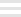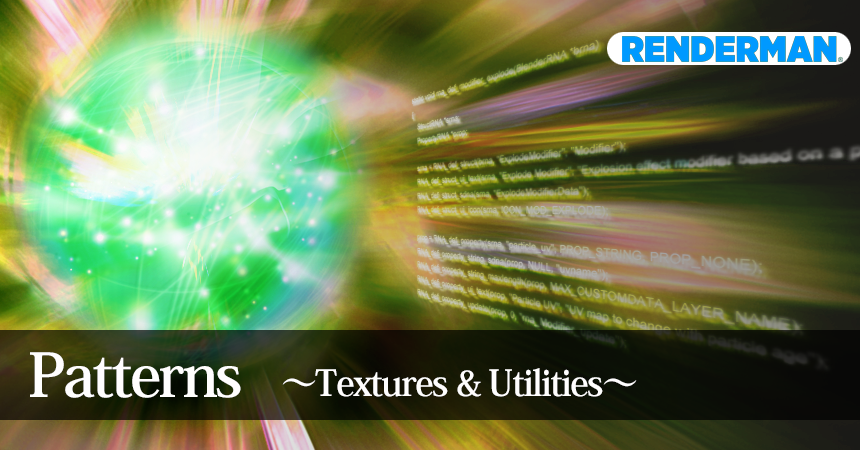#3D Daring Dignified Art

#3D Daring Dignified Art# PxrPhasorNoisehttps://rmanwiki.pixar.com/display/REN/PxrPhasorNoise

### PxrFractal Attributes

• inputManifold
座標の移動
• Dimensions
2D Texture or 3D Texture
• Align Mode
基本軸を決める
• Frequency
波形の調整
• Frequency Flatten
オブジェクトの法線に反して波形調整

### Shaping

• Shaping Mode
波形のデザイン（模様）
• Shaping Soften
背景模様をぼかす。

### Direction

• Direction
波形（XYZ軸）の方向
• Direction Align
Align Modeに準拠し、linear, cylindrical, or spherical パターンに調整。
• Direction Jitter
波形の方向をずらす。
• Direction Jitter Frequency
波形の方向をずらしに調整（ノイズを入れる）
• Direction Jitter Scale
XYZ軸のScale
• Direction Rotate
XYZ軸のRotate
• Direction Flatten
オブジェクトの法線に反して波形方向を調整
• Phase Offset
波形のオフセット
• Phase Align
Align Modeに準拠し、波形のノイズ量を減らす。

### Kernel（中核）

• Kernel Frequency
波形各1本の形の調整
• Kernel Scale
XYZ軸のScale
• Kernel Neighbors
How many near kernel neighbors to sample for phasor waves to convolve. In 2D, you access (2n+1)^2 neighboring kernels. In 3D, you access (2n+1)^3 neighboring kernels. With the default of 2, that is 25 kernels for 2D and 125 kernels for 3D! More kernels are more expensive but give potentially smoother results.
• Kernel Impulses
How many impulses to sample per phasor wave kernel. They are randomly scattered within each phasor wave kernel grid cell, but given a uniform distribution of phase offsets. More impulses are more expensive.
• Kernel Falloff
Each phasor wave kernel has a cosine shaped falloff from its center. This control is a power function on the falloff, decreasing will flatten the area of influence, increasing will sharpen the area of influence.
• Kernel Error
Set how much error and discontinuity is allowable in sampling the kernels. At zero we use a cosine falloff to make sure there are no discontinuities, but it's possible with low kernel neighbors and a stretched kernel scale to find areas filled with grey. Increasing above zero switches to a Gaussian falloff to fill in those areas, but can also introduce discontinuities along the kernel cell grid.

### Octaves

• Phasor Octaves
波形をオクターブ上げる。形が大きく変わる。
• Phasor Octave Scale
XYZ軸のScale
• Phasor Octave Weight
The weight of each successive phasor octave.
• Phasor Octave Offset
The phase offset of each successive phasor octave.
• Phasor Octave Rotate
The rotation around the surface normal for each successive phasor octave.
• Fractal Harmonic Mode
The combination mode of each fractal and harmonic octave. These octaves are combined in amplitude space after the phasor result has been evaluated.
• Fractal Octaves
Increasing fractal octaves computes phasor results at different frequencies which are then combined in amplitude space. These octaves will most likely not be aligned with each other.
• Fractal Octave Scale
The frequency scale of each successive fractal octave.
• Fractal Octave Weight
The weight of each successive fractal octave.
• Fractal Octave Offset
The phase offset of each successive fractal octave.
• Fractal Octave Rotate
The rotation around the surface normal for each successive fractal octave.
• Harmonic Octaves
Increasing harmonic octaves computes phasor results at exact 2x, 4x, etc. frequency which is then combined in amplitude space. These octaves will be aligned with each other.
• Harmonic Octave Weight
The weight of each successive harmonic octave.
• Harmonic Octave Offset
The phase offset of each successive harmonic octave.